Game theory is increasingly relevant in reinforcement learning where we have multiple agents. Understand the concept of Nash Equilibrium.
Game Theory

## Game Theory¶

• Mathematics of conflict.
• Multiple agents.
• Used in economics.
• Increasingly a part of AI/ML.

1. Two-player zero-sum finite deterministic game of perfect information

•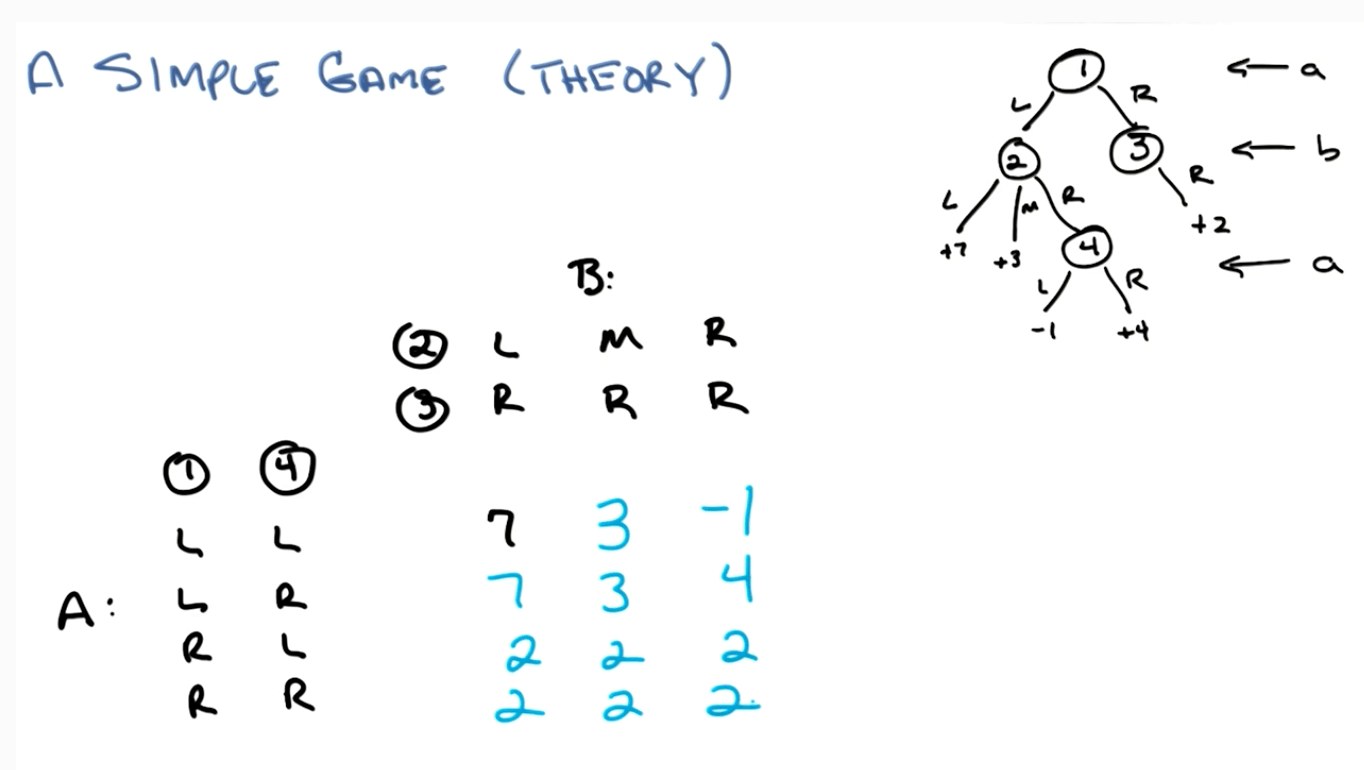• A's points: leaves.
• B's points: negative of A.
• Pure strategy: 2
• A: maximize
• B: minimize

Minimax $\equiv$ Maximax Strategy

• Both A and B would consider the worst case counter strategy.
• A: maximize points.
• B: minimize points.
• There would always have an optimal pure strategy for each player.
• You are assuming they are doing the same thing.
• You are also assuming they are assuming everyone else are doing the same thing.

2. Two-player zero-sum finite non-deterministic game of perfect information

•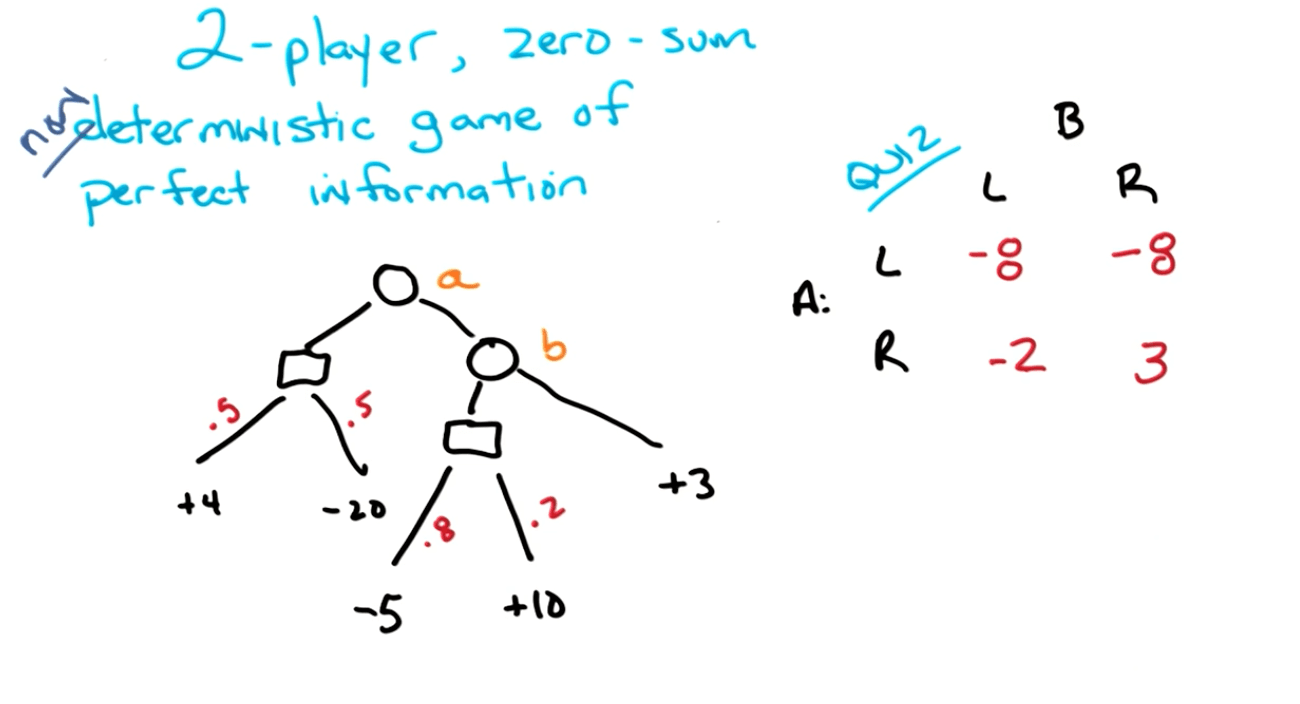• Pure Strategy: -2
• A would maximize
• B would minimize

3. Two-player zero-sum finite non-deterministic game of hidden information

• Mini-poker
• A is dealt a card, red or black with a probability of 50% each
• Red is bad for A
• Black is good for A
• A may resign if given a red card: - 20 for A
• Else A can hold:
• B resigns: + 10
• B sees:
• If red: - 40
• If black: + 30
•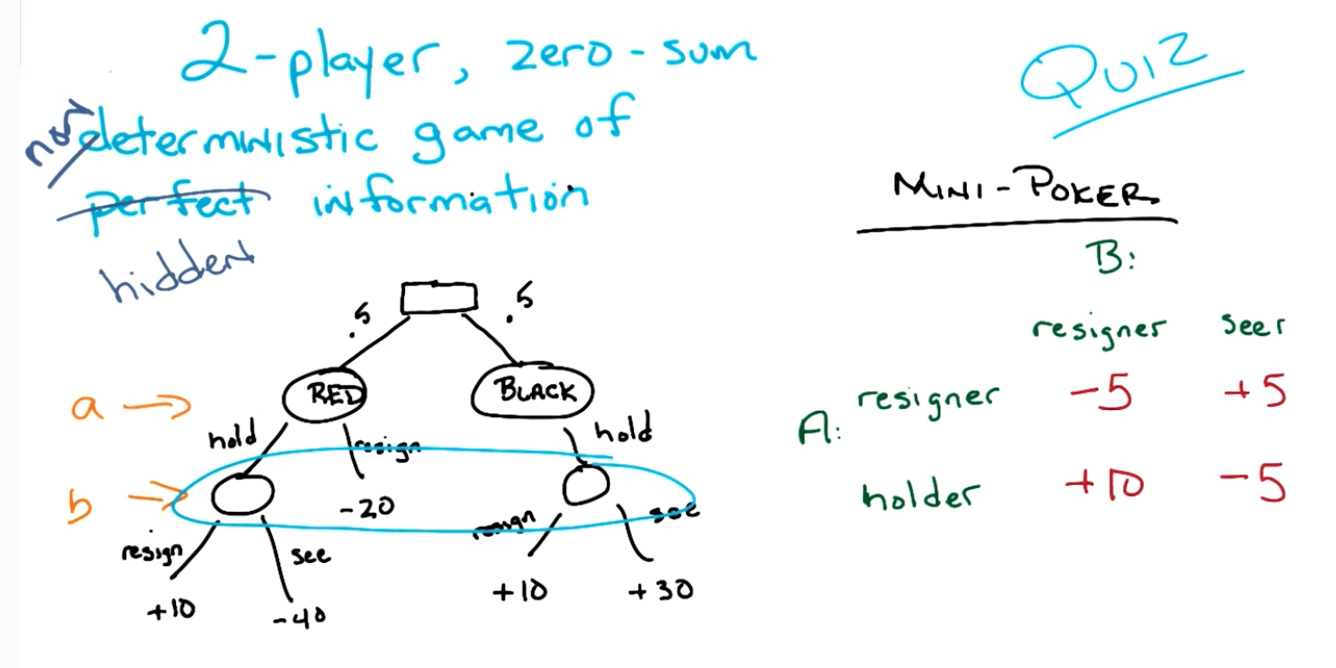• Minimax is not equal to Maximax in this scenario.
• A's strategy depends on what B would do.
• B's strategy depends on what A would do.
• We can use a mixed strategy here.
• There's a distribution over strategies.
• Assume p is the probability of being a "holder" and A has a mixed strategy.
• B: resigner
• A's expected profit:
• 10p + (1-p)(-5) = 15p - 5
• B: seer
• A's expected profit:
• -5p + (1-p)(5) = -10p + 5
• We equate the equations to find out that we have p=0.4, where the expected value of game is +1.
•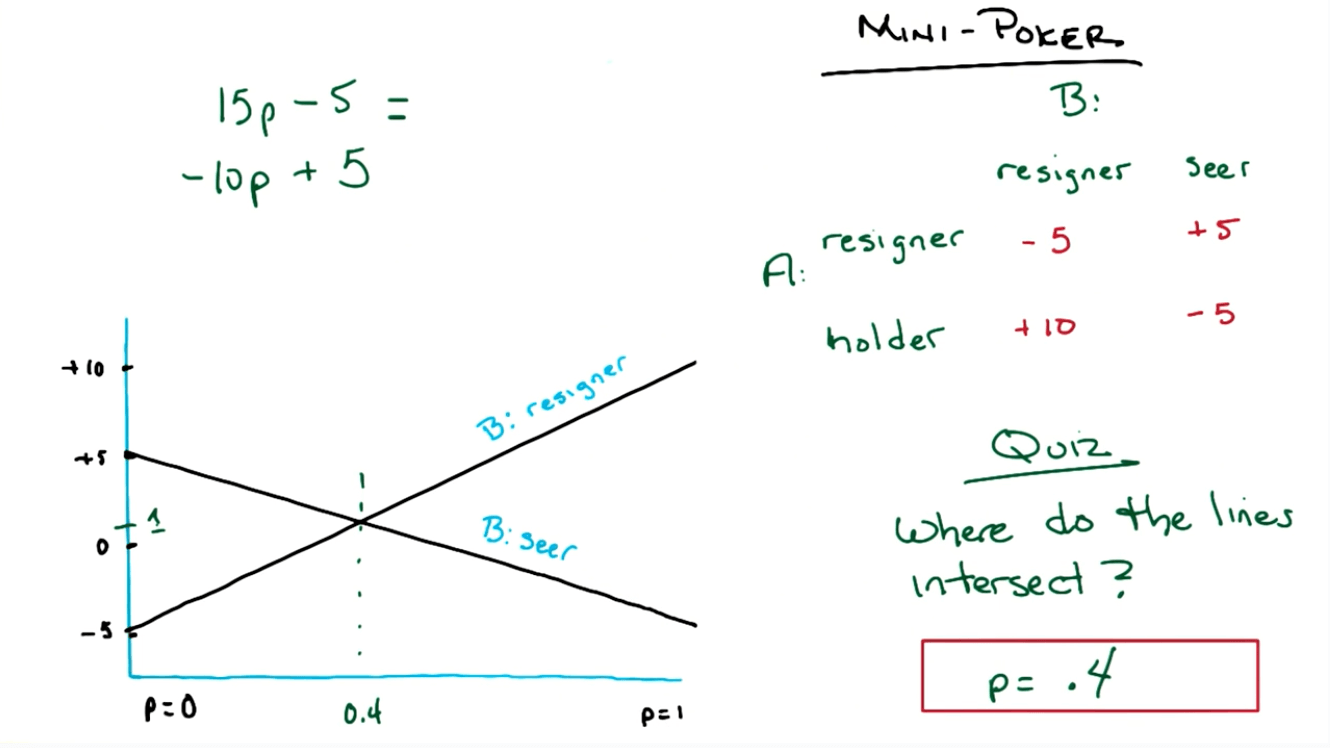4. Two-player non-zero-sum finite non-deterministic game of hidden information

• Typical scenario where the district attorney offers a deal to both criminals to turn on one another.
•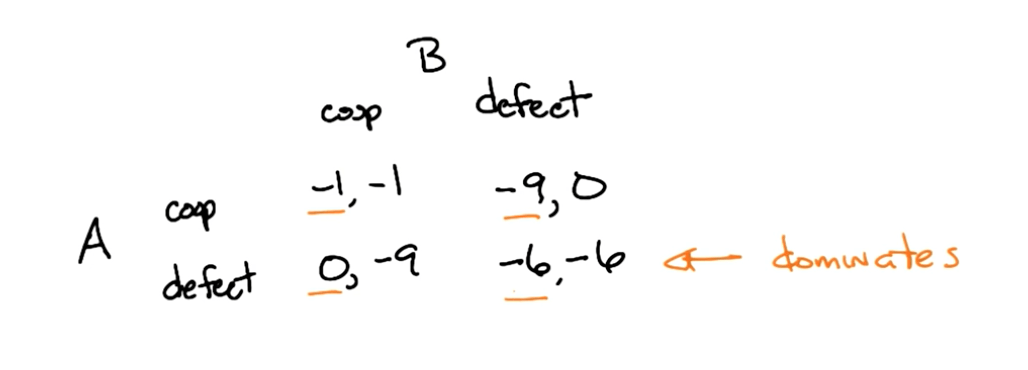• No matter the response of the other prisoner, both would choose to defect. There is a dominance of a particularly strategy here.
• This is also known as the Prisoner's Dilemma.

Nash Equilibrium

• n players with strategies $S_1, S_2 ... S_n$
• $S^*_1 \in S_1 ... S^*_n \in S_n$ are in Nash Equilibrium if
• $\forall_i \ \ S^*_i = argmax_{s_i} utility_i(S^*_1 ... S^*_n)$ there is no reason for anyone to change.

Nash Equilibrium Notes

• In the n-player pure strategy game, if elimination of strictly dominated strategies elimates all but one combination, that combination is the unique NE.
• Any NE will survive elimination of strictly dominated strategies.
• If n is finite and for all strategies which are finite, then there exist at least one NE.
• If you have n-repeated game, you have n-repeated NE.